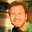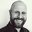Question-and-Answer Resource for the Building Energy Modeling Community
Get started with the Help page

call an idf object field value for use in another object fieldI want to dynamically assign the Vertex values of the following surface

Shading Surface 1, !- Name , !- Transmittance Schedule Name , !- Number of Vertices 0, 2.5, 4.572, !- X,Y,Z Vertex 1 {m} 0, 0, 4.572, !- X,Y,Z Vertex 2 {m} 1.22, 0, 4.572, !- X,Y,Z Vertex 3 {m} 1.22, 2.5, 4.572; !- X,Y,Z Vertex 4 {m}

based on some calculations that include the latitude of the site.

Site:Location, Tampa International Ap, !- Name 27.97, !- Latitude {deg} -82.53, !- Longitude {deg} -5, !- Time Zone {hr} 6; !- Elevation {m}

I would like to call the 27.97 latitude value. Any tips? The following example is useful but not exactly what I am looking for: Group Parametrics

Thank you.

edit retag close merge delete

You need something that works outside of the IDF file. Like an OpenStudio Measure or Eppy.

Sort by » oldest newest most votedAs @__AmirRoth__ says, you need something outside of EnergyPlus to do this. That could be Eppy, as in the script below.

from eppy.iddcurrent import iddcurrent
from eppy.modeleditor import IDF
from future.moves.itertools import zip_longest
from six import StringIO

iddsnippet = iddcurrent.iddtxt
iddfhandle = StringIO(iddcurrent.iddtxt)
IDF.setiddname(iddfhandle)

coords = surface.coords

# do what you want with the coords here

# make the coords into a flattened list
coords = [i for point in coords for i in point]
# find the vertex fields
n_vertices_index = surface.objls.index('Number_of_Vertices')
last_item = len(surface.obj)
first_item = n_vertices_index + 1 # X of first coordinate
vertex_fields = surface.objls[first_item:last_item]

# set the vertex field values
for field, x in zip_longest(vertex_fields, coords, fillvalue=""):
surface[field] = x

idf = IDF()
latitude = idf.getobject('SITE:LOCATION', 'Tampa International Ap').Latitude

idf.save('my_new.idf')
more

1